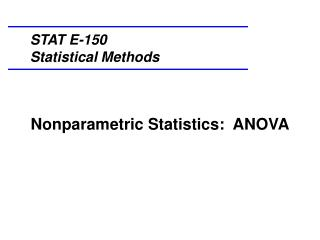DownloadDownload PresentationNonparametric Statistics: ANOVA

# Nonparametric Statistics: ANOVA

Download Presentation## Nonparametric Statistics: ANOVA

- - - - - - - - - - - - - - - - - - - - - - - - - - - E N D - - - - - - - - - - - - - - - - - - - - - - - - - - -
##### Presentation Transcript

1. STAT E-150Statistical Methods Nonparametric Statistics: ANOVA

2. The nonparametric analog to ANOVA is the Kruskal-Wallis Test, which tests the hypothesis that all samples were drawn from populations with the same shape. As with other nonparametric tests, there is no requirement that the residuals are normally distributed.

3. Like the Mann-Whitney Test, the Kruskal-Wallis test ranks all of the observed values. If there are differences between the groups, then the values for each group will be clustered within the ranked data. If there are no differences between the groups, the values will be intermixed within the ranked data. The null hypothesis says that there are no differences among the groups, and so the data values will not be clustered within the ordered data.

4. Start by ranking all scores without regard to their groups, and then find the ranks for each group. The sums are denoted by Ri. If the null hypothesis is true, we would expect all values of Ri to be about equal, assuming that the sample sizes are all equal. A measure of the degree to which the Ri differ from each other is Where k = the number of groups Ni = the number of observations in the ith group Ri = the sum of the ranks in the ith group N = the total sample size

5. Suppose that we would like to investigate whether the number of simple arithmetic problems solved correctly in one hour is different for subjects given a depressant drug, a stimulant drug, or a placebo. Here is the data:

6. Note that in the Depressant group, three of the subjects were able to do very little, whereas in the Stimulant group, three of the subjects answered all questions correctly.

7. The histograms, with Normal curves superimposed, indicate that the data is nonnormal:

8. We will use SPSS to find the results: The mean ranks of the three groups appear different, but we will use a statistical test to see if this is correct.

9. We will use SPSS to find the results: H0: μd = μs = μp Ha: The means are not all equal The Kruskal-Wallis statistic is 2 = 10.407 with p = .005 and 3 - 1 = 2 df. Since p is small, the null hypothesis is rejected. The data indicates that there is a significant difference between the groups.

10. We will use SPSS to find the results: In context, the data indicates that the number of simple arithmetic problems solved correctly in one hour is different for subjects given a depressant drug, a stimulant drug, or a placebo.

11. Using SPSS Note that the groups are in one column and the data is in another:

12. To create the histograms: Click on > Graphs > Chart Builder and choose Histogram. Drag the response variable to the horizontal axis and click on the Groups/Point ID tab.

13. Choose Columns panel variable.

14. Then drag the grouping variable to the Panel box.

15. To add the Normal curves, click on Element Properties, then select Display Normal curve and Apply. Then click on OK.

16. For the Kruskal-Wallis test: Click on > Analyze > Nonparametric Tests > Legacy Dialogs > K Independent Samples Choose the Test Variables and the Grouping variable. Select Kruskal-Wallis H as the Test Type

17. Click on Define Range… and enter the minimum and maximum values for the grouping variable. Click on Continue and then on OK.

18. Here are the results: